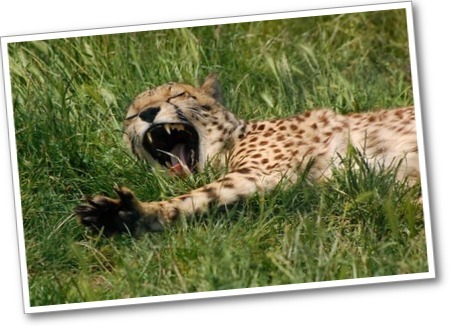# Gallery Problems# Represent a Math Problem

1. Explore each of these math representations: a number line and a double number line.

• Select one representation to describe to the class.
2. Solve this problem. Use a number line or a double number line to help you solve it.

The cheetah at the zoo has a mass of 45 kg. This is 5 times the mass of the fisher cat.

• How many more grams is the mass of the cheetah than of the fisher cat?
3. The cheetah at the zoo has a mass of 45 kg. This is 5 times the mass of the fisher cat. How many more grams is the mass of the cheetah than of the fisher cat?

Mia says:

“The mass of the cheetah is 36 g more than the mass of the fisher cat.”

• Is Mia’s answer reasonable? Explain.
1 of 3